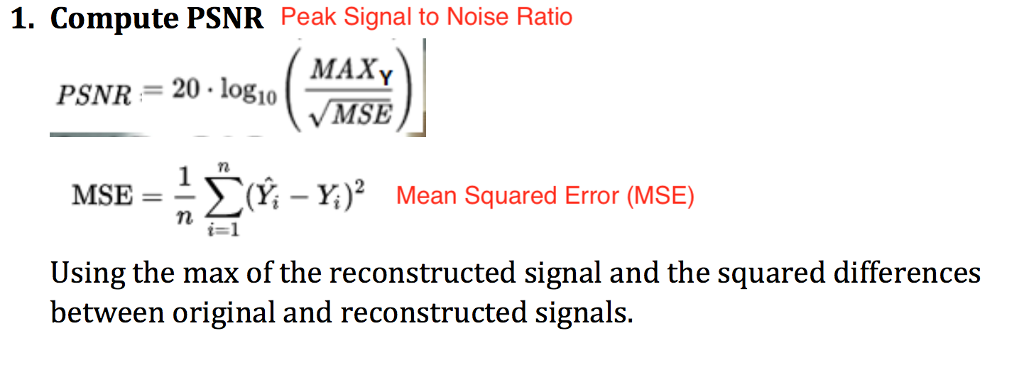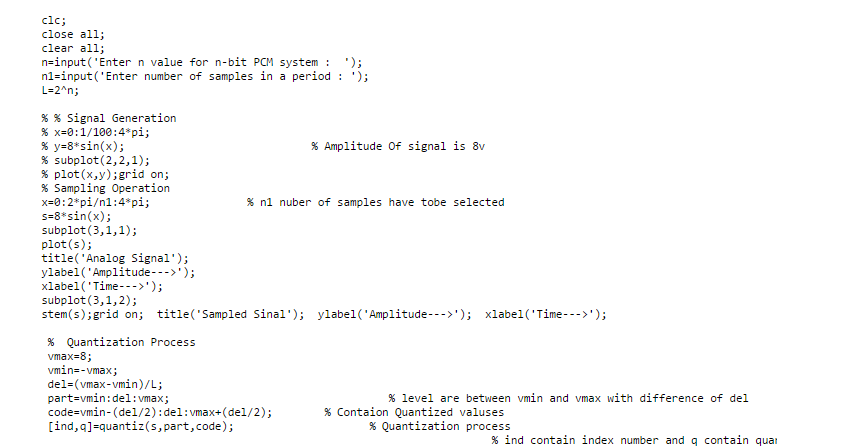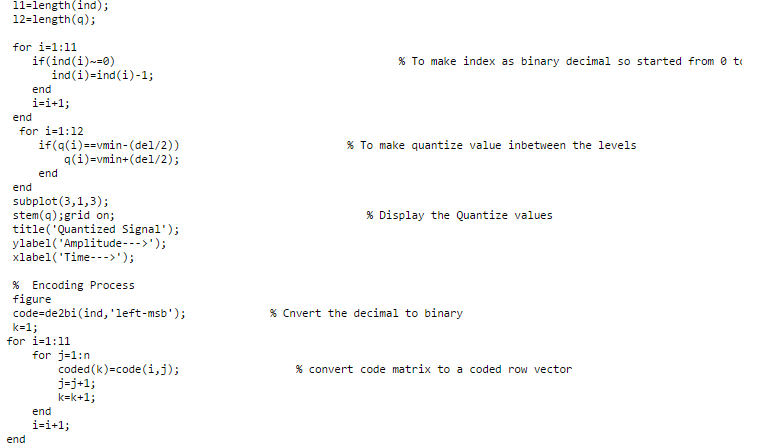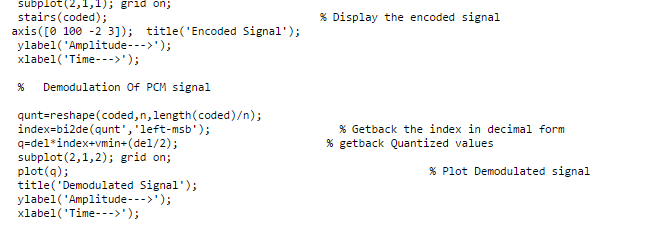# Answered! Using matlab to solve equation PCM for Sound Processing…

Using matlab to solve equation

PCM for Sound Processing

Don't use plagiarized sources. Get Your Custom Essay on
Answered! Using matlab to solve equation PCM for Sound Processing…
GET AN ESSAY WRITTEN FOR YOU FROM AS LOW AS \$13/PAGE% ============

% PCM ENCODING

% ============

% from https://www.mathworks.com/matlabcentral/fileexchange/34610-pcm-matlab-code/content/Untitled.m

% modified by JEC for CSE408 HW3

clc;

clear all;

f = 2; % input frequency 2Hz

fs = 20; % sampling at 20Hz

Ts = 1/fs;

fss = 1.e4; % fine sampling for the time axis 10k sampls/sec

Tss = 1/fss;

t = 0:Tss:2-Tss; % continuous time axis, 2 seconds, 20k samples

d = Ts/40:Ts:2+Ts/40; % discrete time axis 41 samples starting at…

% a very small value, 1/40 of the Sampling Period

% rectangular pulses 1/Ts wide are 20 samples (1/fs*25)/(1/Tss)) wide in the t scale (which

% is 10000Hz, while fs is 20Hz

p = pulstran(t,d,’rectpuls’,1/(fs*25));

% =================

% Analog MSG Signal

% =================

% simple sinusoid at f (2fps) with a +shift of 1.1 to keep all values above

% zero

m = sin(2*pi*f*t)+1.1; % constant 1.1 is added so all values are positive

% HW part 1

% comment the line above (and assign a new value to it)

% add 1Hz 25% amplitude modulation to the input m, i.e. positive

% amplitude variation between 0.75 and 1.25

% Hint: the modulating sin or cos amplitude is 0.25, so you need to add 1.0

% observe the reconstructed signal, why is it not correct?

% what adjustment do you need to do to the signal so the dynamic range

% remains as before?